# fx-350ES PLUS-2

Close

• The colors may differ slightly from the original.

## fx-350ES PLUS-2

Number of Functions : 252
Natural textbook display
Display expression same as textbook.
LR44 × 1

## Features

### Simple and easy to use

Optimal education tools realized through s design that emphasizes comfort in the hand, legibility, and usability through the pursuit of simplicity

### Natural Textbook Display

Features Natural Textbook Display format for displaying equations and calculation result just as they appear in textbooks

### Authentic quality

Excellent quality and authentication ensure confidence and peace of mind for use in educational settings

### Main functions

• Prime factorizations
• Random integers
• Fraction calculations
• Combination and permutation
• Statistics (List-based STAT data editor, standard deviation, regression analysis)
• 9 variables
• Table function
• Comes with new slide-on hard case
• #### Coordinate transformation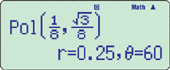• #### Power calculation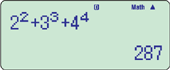#### Statistics

•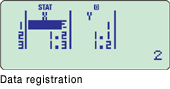•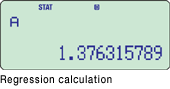•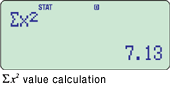#### Trigonometry

•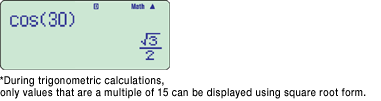#### Table function

•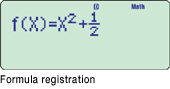•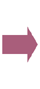•••••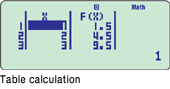## Specifications

• Non Programmable

•Number of Functions : 252

•Natural textbook display Display expression same as textbook.

•LR44 × 1

•10 + 2 digits 10-digit mantissa + 2-digit exponential display.

•Dot matrix display High-resolution screen provides beautiful looking graphs every time.

•Multi-replay Quick and easy recall of previously executed formulas for editing and re-execution.

•Plastic keys Designed and engineered for easy operation.

• Basic Mathematical Functions Trigonometric, Exponential logarithmic, etc.

•List based STAT-data editor Viewing and editing of input data in list format, showing data groups (x-data, y-data, frequency) and surrounding data.

• Basic Statistics Standard statistics functions such as Mean, SUM, Standard Deviation, and Regression

• Table Generation You can create a numerical table based on that expression by registering a function expression. In addition, graph functions can be represented by graphs.

• AAA-size Battery

• Non Graphing

### Size of case / Total weight

• Dimensions (D × W × H) : 161.5×77×11.1mm
• Weight : 95g